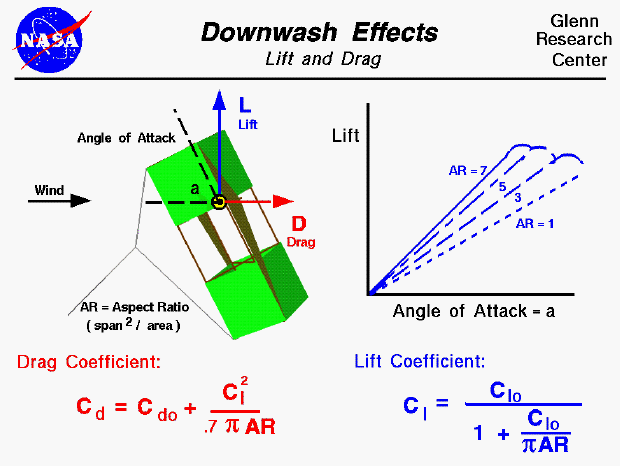An excellent way for students to gain a feel for aerodynamic forces is to fly a kite. Kites can fly because of forces acting on the parts of the kite. Though kites come in many shapes and sizes, the forces which act on the kite are the same for all kites. You can compare these forces to the forces that act on an airliner in flight and you will find that, with the exception of thrust, they are exactly the same. Since the forces on a kite are the same as the forces on an airplane, we can use the mathematical equations developed to predict airplane performance to predict the aerodynamic performance of a kite.

The aerodynamic force on a kite is broken down into the lift which acts perpendicular to the wind direction and the drag which acts along the wind direction. There are several factors that affect the magnitude and the direction of the aerodynamic force. On this page we show the effects of downwash on the lift and drag. The graphic shows a side view of the flying kite with the aerodynamic lift shown by the blue vector, and the drag by the red vector. The wind is blowing parallel to the ground; The kite is inclined to the wind at an angle of attack, a, which affects the amount of lift and drag generated by the kite. Other factors affecting the lift and drag include the wind velocity, the air density.

For any object, the lift and drag depend on the lift coefficient, Cl, and the drag coefficient, Cd of the object. These coefficients are usually determined experimentally for aircraft, but the aerodynamic surfaces for most kites are simple, thin, flat plates. So we can use some experimental values of the lift and drag coefficients for flat plates to get a first order idea of our kite performance. For a thin flat plate, the effects of angle of attack are easily determined. The lift coefficient Clo is equal to 2.0 times pi (3.14159) times the angle a expressed in radians (180 degrees equals pi radians):

Clo = 2 * pi * a

The drag coefficient Cdo is equal to 1.28 times times the sine of the angle a:

Cdo = 1.28 * sin(a)

We use Clo for the lift coefficient and Cdo for the drag coefficient because there is another aerodynamic effect present on most kites. If we think of a kite as an aircraft wing, and use the terminology associated with aircraft wings, most kites have a low wing span (length from side to side) relative to the surface area. Most kites therefore have a low aspect ratio which is defined to be span squared divided by the area. Near the tips of a wing the flow spills from the under side to the top side because of the difference in pressure. This creates a downwash which changes the effective angle of attack of the flow over a portion of the wing. For low aspect ratio wings, the portion of the wing affected by the downwash is greater than for high aspect ratio wings. Since most kites have a low aspect ratio AR, we have to include the effect of the downwash on the lift and drag coefficient. The equation for the lift coefficient is:

Cl = Clo / (1 + Clo / (pi * AR) )

The effect of downwash on lift coefficient is shown on the plot. Low aspect ratio wings (AR ~ 1) have significantly less lift than higher aspect ratio wings (AR ~7). The effect of downwash on the drag coefficient is called the "drag due to lift" or the induced drag of the wing. The equation for induced drag is:

Cd = Cdo + Cl^2 / (.7 * pi * AR) )

where the .7 is an efficiency factor for rectangular wing shapes.

With these equations you can make a first prediction of the lift and drag of your kite. You can use the KiteModeler program to further study how kites work and to design your own kites.Beginner's Guide to Aerodynamics
Beginner's Guide to Propulsion
Beginner's Guide to Model Rockets
Beginner's Guide to Kites
Beginner's Guide to Aeronautics